# ISEE Middle Level Math : How to find a ratio

## Example Questions

← Previous 1 3

### Example Question #1 : How To Find A Ratio

What is the least common multiple of 5, 12, and 15?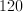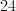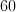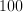Explanation:

You can write out the multiples of each number, and find the first number that is common to all of them.

5: 5, 10, 15, 20, 25, 30, 35, 40, 45, 50, 55, 60

12: 12, 24, 36, 48, 60

15: 15, 30, 45, 60

### Example Question #1 : Ratio And Proportion

What is the greatest common factor of 12, 24 and 96?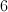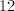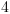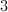Explanation:

To find the greatest common factor of three numbers, first factor each number as a product of prime numbers: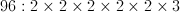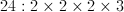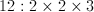Now take all of the factors that are in common between 12, 24 and 96.  In this case, that is 2, 2 and 3.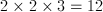### Example Question #3 : How To Find A Ratio

Logan needs to answer 60 questions to finish his test. If he has answered 25% of the questions in 20 minutes, how long will it take him to complete the entire test?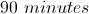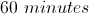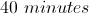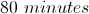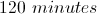Explanation:

To solve:

First, find the number of answers completed by calculating: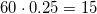Then, find how long it would take him to complete the 60 question test if for every 20 minutes he answer 15 questions: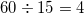(sets of 15 questions)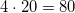minutes altogether to finish the test.

### Example Question #4 : How To Find A Ratio

Which ratio is equivalent to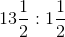?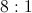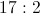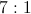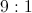Explanation:

A ratio can be rewritten as a quotient; do this, and simplify it.Rewrite as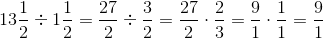or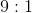### Example Question #5 : How To Find A Ratio

I have 5 white chocolates, 3 milk chocolates, and 9 dark chocolates. What is the ratio of white chocolates to the other types of chocolates?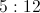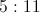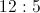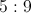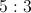Explanation:

There are 5 white chocolates. There are a total of 12 milk and dark chocolates. The ratio of white to other chocolates is 5:12.

### Example Question #6 : How To Find A Ratio

In February, there were 12 rainy days, 6 cloudy days and 10 sunny days. What was the ratio of sunny days in February?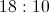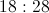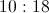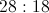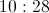Explanation:

Identify the number of sunny days in February :Then, creating a ratio,  show the number of sunny days compared to the total number of days in the month of February (28):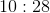Answer:### Example Question #7 : How To Find A Ratio

Express the following ratio in simplest form: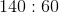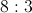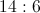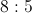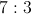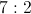Explanation:

Rewrite this in fraction form for the sake of simplicity, and divide both numbers by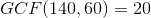: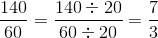The ratio, simplified, is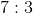.

### Example Question #8 : How To Find A Ratio

Express this ratio in simplest form: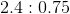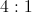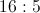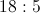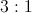Explanation:

A ratio involving decimals can be simplified as follows:

First, move the decimal point over a common number of places in each number so that both numbers become whole. In this case, it would be two places: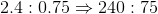(note the addition of a zero at the end of the first number)

Now, rewrite as a fraction and divide both numbers by: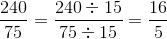The ratio, simplified, is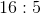### Example Question #9 : How To Find A Ratio

Express this ratio in simplest form: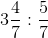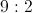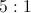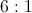Explanation:

A ratio of fractions can best be solved by dividing the first number by the second. Rewrite the mixed fraction as an improper fraction, rewrite the problem as a multiplication by taking the reciprocal of the second fraction, and cross-cancel: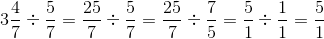The ratio, simplified, is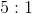.

### Example Question #10 : How To Find A Ratio

Write as a unit rate: 450 words typed in 25 minutes.

23 words per minute

19 words per minute

18 words per minute

17 words per minute

22 words per minute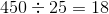words per minute